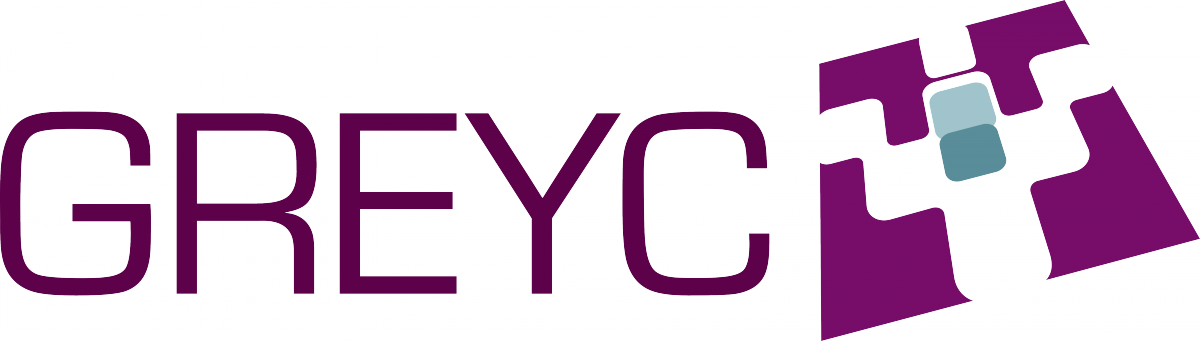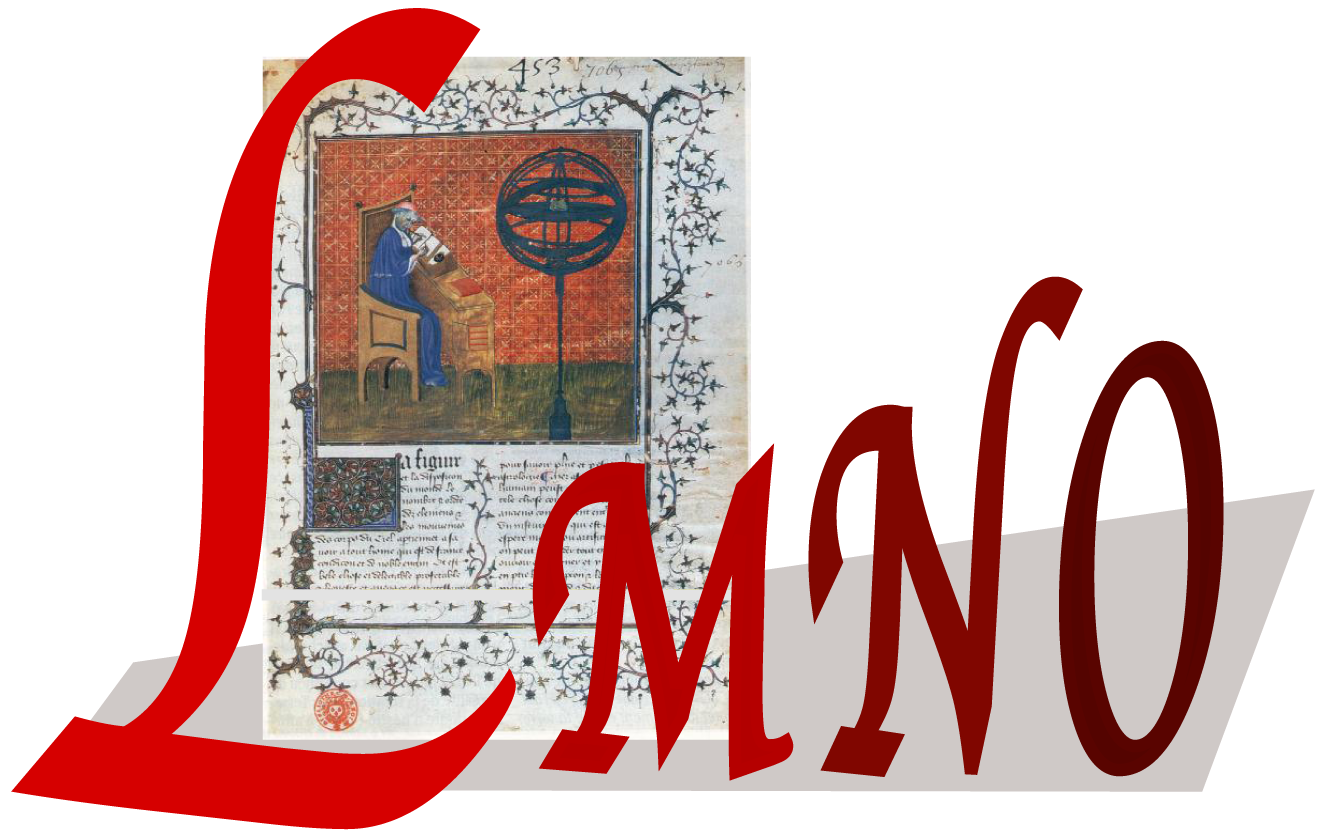Séminaire Cryptologie & Sécurité
Contacts :
À venir :
Private Set Intersection from Homomorphic Encryption: A Python Implementation

Résumé.

Private Set Intersection (PSI) is an interactive protocol between a client and a server. The client holds a set of items X and the server holds a set of items Y. By the end of the protocol, the client learns the intersection of X and Y and nothing else about the server's set, while the server learns nothing about the client's data.

In this presentation, I will first motivate our interest in PSI, by providing a list of applications: private contact discovery for Whatsapp or Signal, measuring ads efficiency privately or DNA pattern matching. Then, I will describe our Python implementation of the PSI protocol described in here and here .

[16 juin 2021 | 14h | BBB (Univ)]
Passé:
Attaques Boomerang et chiffrements de type Feistel

Résumé.

Cet exposé se concentre sur une technique de cryptanalyse appelée l'attaque boomerang, introduite en 1999 par David Wagner et récemment affinée dans plusieurs publications. Nous reviendrons sur la définition de distingueur boomerang et sur les différentes techniques utilisées pour en estimer la probabilité, depuis le découpage dit en sandwich jusqu'aux avancées récentes et l'introduction de la Boomerang Connectivity Table (BCT). Nous montrerons ensuite que la BCT ne s'applique pas directement aux chiffrements de type Feistel et introduirons une nouvelle table, appelée la FBCT, pour pallier ce manque.

[2 juin 2021 | 14h | BBB (Univ)]
A Quick Intro to Searchable Encryption : Theory & Practice - Constructions & Attacks

Résumé.

Introduction rapide aux Algorithmes de recherche sur bases de données chiffrées : théorie et pratique - constructions et attaques.

[21 avril 2021 | 14h | BBB (Univ)]
On algorithms for solving Euclidean lattice problems in cryptography

Résumé.

In this talk, we will try to review the state-of-the-art of the algorithms for solving the Euclidean lattice problems underlying cryptography. In more details, this talk contains two parts. In the first part, we will focus on the lattice problems such as approximate Shortest Vector Problem (approx-SVP) and the lattice reduction algorithms as the best known solving algorithms so far. In particular, I will present an improved enumeration-based lattice reduction algorithm, which is shown to be (potentially) relevant to cryptanalysis. In the second part, we will instead consider a quantum problem that is computationally equivalent to approx-SVP. By directly solving a quantum problem, we may expect to have a more powerful use of the quantum computation. However, the best known algorithms for solving approx-SVP via solving this quantum problem, is not better than lattice reduction yet.

[31 mars 2021 | 14h | BBB (Univ)]
Recovering short secret keys of RLCE in polynomial time

Résumé.

The security of most modern public key encryption algorithms (such as RSA) relies on arithmetic problems. Today, the hardness of these problems is threatened by the potential emergence of large quantum computers. For this reason, cryptographers try to come up with new cryptographic schemes relying on families of problems which remain hard to solve even with a quantum computer. One possible solution is to use the hardness of decoding a random error-correcting code. This field is known as code-based cryptography. This idea was introduced by McEliece in 1978 and his proposal is still considered secure today. However, McEliece's scheme needs large public keys (about 1MB for 256 security bits), which makes it unfit for most use-cases. Therefore, there are several attempts to replace the Goppa codes, used by McEliece, with other families of codes, to obtain shorter keys. In this work, we analyze a proposal from Wang, named Random Linear Code-based Encryption (RLCE), and conclude that for all the short key parameters proposed by the author, we can recover the secret key in polynomial time, by using the dimension of the square code as a distinguisher. This is a joint work with Alain Couvreur and Jean-Pierre Tillich.

[10 mars 2021 | 14h | BBB (Univ)]
Authentification forte par prise d'empreinte de navigateurs

Résumé.

L'authentification web consiste à vérifier que le visiteur d'un site web est bien le détenteur d'un compte. Pour ce faire, plusieurs informations peuvent servir de preuve de détention, dont les empreintes de navigateur. Celles-ci sont des propriétés collectées à partir d'un navigateur permettant d'en constituer une empreinte potentiellement unique. Au travers de cette présentation, nous présentons nos travaux portant sur l'adéquation de ces empreintes pour de l'authentification, l'évaluation de ces dernières selon des propriétés d'informations biométriques, et la proposition d'une méthode de sélection d'attributs tels qu'ils satisfont un niveau de sécurité et réduisent les contraintes d'utilisation des empreintes.

[24 février 2021 | 14h | BBB (Univ)]
The Power Error Locating Pairs algorithm

Résumé.

In this talk we present an overview of some decoding algorithms for Reed-Solomon codes, together with a ``power'' extension of the Error Correcting Pairs algorithm.

It is known that several algorithms have been designed in order to decode Reed-Solomon codes. In particular Welch-Berlekamp algorithm and the Error Correcting Pairs algorithm are two classical algorithms which correct up to half the minimum distance of the code.

Some extensions of Welch-Berlekamp algorithm can even correct beyond this bound: on the one hand two such extensions are Sudan algorithm and Guruswami-Sudan algorithm, which are deterministic and return the list L of all possible solutions (note that in the typical situation |L|=1). On the other hand, the Power Decoding algorithm has the same decoding radius as Sudan algorithm, is quicker but may fail (it gives one solution or zero).

The Error Correcting Pairs algorithm behaves slightly differently with respect to Welch-Berlekamp algorithm, since it mainly consists in localizing errors. After that, decoding boils down to elementary linear algebra. The advantage of this algorithm is that it can be applied to a larger class of codes (Reed-Solomon, algebraic-geometry codes, some cyclic codes). The idea that motivated our work was then to design an extension of the Error Correcting Pairs algorithm with the same versatility and with decoding radius beyond half the minimum distance.

This extension takes the name of Power Error Locating Pairs algorithm. It consists, as for the Power Decoding algorithm, in considering more subproblems (each of them given by a power of the main one) at the same time. Though, while for the Power Decoding algorithm the condition to have a solution depends on the dimension of a linear system solution space, for the Power Error Correcting Pairs algorithm, it depends on the nullity of a certain space.

[10 février 2021 | 14h | BBB (Univ)]
Assessing residual security of lattice-based cryptography

Résumé.

This talk will present a framework for cryptanalysis of lattice-based schemes, when side information —in the form of «hints»— about the secret is available. This presentation outlines a joint work with Dana Dachman-Soled, Léo Ducas and Huijing Gong that was presented in CRYPTO 2020 (EPrint on IACR).

This framework generalizes the primal lattice reduction attack, and allows the progressive integration of hints before running a final lattice reduction step. The techniques for integrating hints include sparsifying the lattice, projecting onto and intersecting with hyperplanes, and/or altering the distribution of the secret vector. The main contribution is to propose a toolbox and a methodology to integrate such hints into lattice reduction attacks and to predict the performance of those lattice attacks with side information.

While initially designed for side-channel information, this framework can also be used in other cases. For example, one can simply exploit constraints imposed by certain schemes (LAC, Round5, NTRU). Besides, I will present a way to use this framework combined with decryption failures information using a joint work with Jan-Pieter D’Anvers and Fernando Virdia presented in EUROCRYPT 2020 (EPrint on IACR).

[27 janvier 2021 | 14h | BBB (Univ)]
Recent Approaches of Speaker Anonymization Techniques

Résumé.

An increasing number of smart devices embed speech-commands. The usage of speech offers simplicity, accessibility and it also opens new human-computer interactions. However, the gathering and exploitation of this type of data raise many privacy threats as speech data is sensitive in nature. Personal information about the speaker can be inferred (e.g., gender, emotion...). In addition, speech is a biometric characteristic, it can be used to identify speakers. To address this issue anonymization techniques have been proposed.

In this talk, we are going to present the recent approaches for speech anonymization techniques with a focus on x-vector based techniques. The evaluation is presented with various scenarios against attackers.

[13 janvier 2021 | 14h | BBB (Univ)]
Making (near) Optimal Choices for the Design and Cryptanalysis of Block Ciphers

Résumé.

One of the many ways to build encryption algorithm in symmetric cryptography is to design what's called a block cipher : a family of permutations indexed by a key, such that choosing a key select one permutation, but it's hard to find the key for a given permutation. The encryption of a given plaintext is then simply its image through the chosen permutation. When designing block ciphers, we need to make decisions on which specific components to use (e.g. S-boxes, linear layer etc.). These decisions are made by taking into account the security of the resulting block cipher, but also the underlying cost in term of performances. In this talk, I will present two different works aiming at finding optimal components w.r.t a given criteria, while also trying to keep a very cheap implementation cost. I will show how the use of automatic tools (either already existing or specifically designed) can help us to make (near) optimal decisions while the initial search space is very large.

After an general introduction to present the context in more details, I will first focus a block cipher construction called Generalized Feistel Networks, which is a very efficient construction in general. The idea of this construction is to split the plaintext into an even number of block, apply a Feistel transformation in parallel to those blocks, and then permute them. In a recent paper accepted at FSE 2020, we showed how to choose this permutation to be optimal w.r.t a certain criterion (called "diffusion round"), which also allowed us to solve a 10-year old problem.

I will finish with an overview of two other works using automatic tools for the design and cryptanalysis of block ciphers as well as a brief idea of my future work.

[2 décembre 2020 | 14h | BBB (Univ)]